RS Aggarwal Solutions Class 10 Ex 18E

RS Aggarwal Class 10 Ex 18E Chapter 18

Q.1: In a given figure, from a rectangular region ABCD with AB = 20 cm, a right triangle AED with AE = 9 cm and DE = 12 cm, is cut off. On the other end, taking BC as diameter, a semicircle is added on outside the region. Find the area of the shaded region.

[ $\pi = 3.14$ ]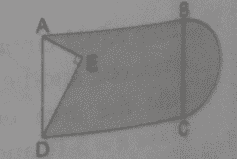Sol:

In right triangle AED $AD^{2} = AE^{2} +DE^{2}$

= $= 9^{2} + 12^{2}$ = 81+144 = 225

$Therefore, AD^{2} = 225$

$\Rightarrow AD = 15$

Area of the shaded region =  Area of rectangle – Area of triangle AED + Area of semicircle

$= AB \times BC – \frac{1}{2} \times AE \times DE + \frac{1}{2} \pi \left ( \frac{BC}{2} \right )^{2}$

$= 20 \times 15 – \frac{1}{2} \times 9 \times 12 + \frac{1}{2} \times 3.14 \left ( \frac{15}{2} \right )^{2}$

$= 300 – 54 + 88.31$

= 334.31 $cm^{2}$

Hence, the area of shaded region is 334.31 $cm^{2}$

Q.2: In the given figure, O is the centre of the circle with AC= 24 cm, AB = 7 cm and $\angle BOD = 90^{\circ}$. Find the area of shaded region. [$\pi = 3.14$]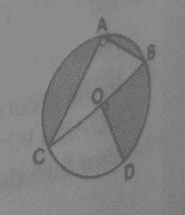Sol:

In right triangle ABC

$BC^{2} = AB^{2} +AC^{2}$

$= 7^{2} +24^{2}$ = 49 + 576 = 625

$Therefore, BC^{2} = 625$

$\Rightarrow BC = 25$

Now, $\angle COD +\angle BOD = 180^{\circ}$      ( Linear pair angles )

$\Rightarrow \angle COD = 180^{\circ} – 90^{\circ} = 90^{\circ}$

Now, area of the shaded region = Area of sector having central angle $360^{\circ} – 90^{\circ}$ – Area of triangle ABC

$= \frac{270}{360}. \pi \left ( \frac{BC}{2} \right )^{2} – \frac{1}{2} AB \times AC$

$= \frac{3}{4} \times 3.14 \left ( \frac{25}{2} \right )^{2} – \frac{1}{2} \times 7 \times 24$

= 367.97 – 84 = 283.97 $cm^{2}$

Hence, the area of shaded region is 283.97 $cm^{2}$

Q.3: In the given figure, a circle is inscribed in an equilateral triangle ABC of side 12 cm. Find the radius of inscribed circle and the area of the shaded region.  [Use$\sqrt{3} =1.73 \; and \;\pi = 3.14$ ]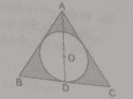Sol:

We can find the radius of the in circle by using the formula

$r = \frac{2\times Area \; of \; triangle}{Perimeter \; of \; triangle } = \frac{2\times \frac{\sqrt{3}}{4}\times (12)^{2}}{3 \times 12} = 2\sqrt{3} cm$

Now area of shaded region = Area of triangle – Area of circle

= $\frac{\sqrt{3}}{4} \times (12)^{2} – 3.14 \times (2\sqrt{3})^{2}$

= 62.28 – 37.68 = 24.6 $cm^{2}$

Hence the area of shaded region is 24.6 $cm^{2}$

Q.4: On a circular table cover of radius 42 cm, a design is formed by a girl leaving an equilateral triangle ABC in the middle, as shown in the figure. Find the covered area of the design. [Use$\sqrt{3} =1.73 \; and \;\pi = 3.14$ ]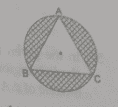Sol:

Construction: Join AO and extend it to D on BC.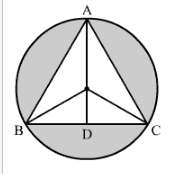Radius of the circle, r = 42 cm

$\angle OCD = 30^{\circ}$

$\cos 30^{\circ}= \frac{DC}{OC}$

$\Rightarrow \frac{\sqrt{3}}{2}= \frac{DC}{42}$

$\Rightarrow DC = 21 \sqrt{3}$

$\Rightarrow BC = 2\times DC = 2\times 21 \sqrt{3} =72.66$ cm

$\sin 30^{\circ} = \frac{OD}{OC}$

$\Rightarrow \frac{1}{2} = \frac{OD}{42}$

$\Rightarrow OD = 21$ cm

Now, AD= AO + OD = 42 + 21 = 63 cm

Area of the shaded region = Area of circle – Area of triangle ABC

$= \pi (OA)^{2} – \frac{1}{2} \times AD \times AB$

$= \frac{22}{7} (42)^{2} – \frac{1}{2} \times 63 \times 72.66$

= 5544 – 2288.79 =3255.21 $cm^{2}$

Q.5: The perimeter of the quadrant of a circle is 25 cm. Find its area.

Sol:

Let the radius of the circle be r.

Now, perimeter of quadrant = $\frac{1}{4} (2 \pi r) + 2r$

$\Rightarrow 25 = \frac{1}{2} \times \frac{22}{7 } \times r +2r$

$\Rightarrow 25 = \frac{25r}{7}$

$\Rightarrow r = 7 cm$

Area of quadrant $= \frac{1}{4} \pi r^{2}$

$= \frac{1}{4} \times \frac{22}{7} \times 7 \times 7 = 38.5\; cm^{2}$

Hence the area of quadrant is $38.5\; cm^{2}$

Q.6: A chord of a circle of radius 10 cm subtends a right angle at the centre. Find the area of the minor segment. [Use $\pi = 3.14$ ]

Sol: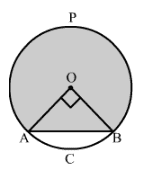Area of minor segment = Area of sector AOBC – Area of right triangle AOB

$\frac{\theta }{360} . \pi (OA)^{2} – \frac{1}{2} \times OA \times OB$

$\frac{90}{360} \times 3.14 (10)^{2} – \frac{1}{2} \times 10 \times 10$

=78.5 – 50 = 28.5 $cm^{2}$

Hence, the area of minor segment is 28.5 $cm^{2}$

Q.7: The radius of a circular garden is 100 m. There is a road 10 m wide, running all around it. Find the area of the road and the cost of levelling it at Rs. 20 per $m^{2}$.  [Use $\sqrt{3} = 1.73$ ]

Sol:

Area of the rod = Area of outer circle – Area of inner circle

= $\pi R^{2} – \pi r^{2}$

$= \pi [(110)^{2} – (100)^{2} ]$

= $3.14 \times 2100$

$= 6594 \; m^{2}$

Cost of levelling the road = Area of the road $\times$ Rate

$= 6594 \times 20$ = Rs. 131880

Q.8: The area of an equilateral triangle is $49 \sqrt{3} \; cm^{2}$. Taking each angular point as centre, circles are drawn with radius equal to half the length of the side of the triangle. Find the area of the triangle not included in the circle. [Taking $\sqrt{3} = 1.73$ ]

Sol:

Area of equilateral triangle = $49 \sqrt{3}$

$\Rightarrow \frac{\sqrt{3}}{4} \times (Side)^{2} = 49 \sqrt{3}$

$\Rightarrow (Side)^{2} = 7^{2} \times 2^{2}$

$\Rightarrow (Side) = 7 \times 2 = 14$ cm

Radius of the circle = Half of the side of the triangle = 7 cm

Area of the triangle not included in the circle = Area of triangle – Area of 3 sectors having central angle $60^{\circ}$

=$49 \sqrt{3} – 3 \times \frac{60}{360} \times \frac{22}{7} \times 7 \times 7$

= 84.77 – 77 = 7.77 $cm^{2}$

Hence, the required area is 7.77 $cm^{2}$

Q.9: A child draws the figure of an aeroplane as shown. Here, the wings ABCD and FGHI are parallelograms, the tail DEF is an isosceles triangle, the cockpit CKI is an semicircle and CDFI is a square. In the given figure, $BP \perp CD$, $HQ \perp FI$ and $EL \perp DF$. If CD = 8 cm, BP= HQ = 4 cm and DE= EF= 5cm, find the area of the whole figure.  [ Use $\pi = 3.14$ ]

Sol:

CD = 8 cm

BP = HQ = 4 cm

DE = EF = 5 cm

Area of the parallelogram ABCD = $B \times H$

= $8 \times 4 = 32 \; sq. \; cm$

Area of the parallelogram FGHI = $B \times H$

= $8 \times 4 = 32 \; sq. \; cm$

Area of the square = $Side^{2}$ $= 8^{2}= 64$ sq. cm

In $\bigtriangleup ELF$, we have :

$EL^{2} = 5^{2} – 4^{2}$ = 9

$EL = 3$ cm

Area of the semicircle = $\frac{1}{2} \times H$

$= \frac{1}{2} \times 8 \times 3$ = 12 s q. cm

Area of the semicircle = $\frac{1}{2} \pi r^{2}$

$= \frac{1}{2} \times \frac{22}{7} \times 16$ = 25.12 sq.cm

Therefore, Total area = Area of the parallelogram ABCD+ Area of the parallelogram FGHI + Area of the traingle DEF + Area of the semicircle CKI + Area of the square

Thus total area = 165.12 $cm^{2}$

Q.10: A circular disc of radius 6 cm is divided into three sector with central angles $90^{\circ}, 120^{\circ} \; and \; 150^{\circ}$. What part of the whole circle is the sector with central angle $150^{\circ}$? Also, calculate the ratio of the three sectors.

Sol:

Area of the sector having central angle = $150^{\circ} = \frac{150}{360}. \pi (6)^{2} = \frac{5}{12} \times Area of circular disc$

Now, Area of sector having central angle $90^{\circ}$ : Area of sectors having central angle $120^{\circ}$ : Area of sector having central angle $150^{\circ}$

= $\frac{90^{\circ}}{360^{\circ}}. \pi (6)^{2} : \frac{120^{\circ}}{360^{\circ}}. \pi (6)^{2} : \frac{150^{\circ}}{360^{\circ}}. \pi (6)^{2}$

= $\frac{1}{4} : \frac{1}{3} : \frac{5}{12}$ = 3:4:5

Q.11: A round table cover has six equal design as shown in the given figure. If the radius of the cover is 35 cm then find the total area of the design. [ $\sqrt{3} = 1.732 \; and \; \pi = 1.732$ ]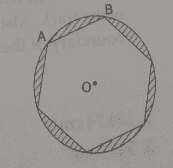Sol: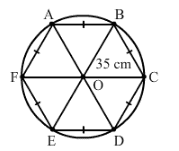Join each vertex of the hexagon to the centre of the circle.

The hexagon is made up of six triangles.

Total area of the design = Area of the circle – Area of the six triangles

$= \left [ \frac{22}{7} \times 35 \times 35 \right ] – \left [ 6 \times \frac{\sqrt{3}}{4} \times 35 \times 35 \right ]$

$= 35 \times 35 \left [ \frac{22}{7} – \left ( \frac{6\times 1.732}{4} \right )\right ]$

$= 1225 \left [ \frac{22}{7} – 2.598 \right ]$

$= 1225 \times 0.542$

$= 663.95 \; cm^{2}$

Q.12: In the given figure, PQ= 24 cm, PR = 7 cm and O is the centre of the circle . Find the area of the shaded region. [ $\pi = 1.732$ ]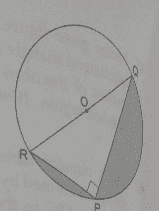Sol:

In the right $\bigtriangleup RPQ$, we have:

$RQ = \sqrt{RP^{2} + PQ^{2}}$

$= \sqrt{7^{2} + 24^{2}}$

$= \sqrt{49 + 576}$ = 25 cm

OR = OQ = 12.5 cm

Now, Area of the circle = $\pi r^{2}$

$= 3.14 \times 12.5 \times 12.5$

$= 490.625$ sq. cm

Area of semicircle = $= \frac{490.625}{2} = 245.31$ sq. cm

Area of triangle = $= \frac{1}{2} \times b \times h = \frac{1}{2} \times 7 \times 24 = 84$ sq. cm

Thus we have:

Area of shaded part = Area of the semicircle – Area of the triangle

= 245.31 – 84 = 161.31 sq. cm

Q.13: In the given figure, $\bigtriangleup ABC$ is right-angled at A. Find the area of the shaded region if AB = 6cm, BC = 10 cm and O is the centre of the incircle of $\bigtriangleup ABC$. [ $\pi = 1.732$ ]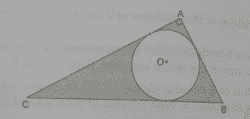Sol:

Using pythagoras theorem for triangle ABC, we have:

$CA^{2} + AB^{2} = BC^{2}$

$CA^{2} = BC^{2} – AB^{2}$

$CA = \sqrt{BC^{2} – AB^{2}}$

$CA = \sqrt{100 – 36}$

$CA = \sqrt{64}$ = 8 cm

Now, we must find the radius of the incircle .Draw OE, OD and OF perpendicular to AC, AB and BC respectively.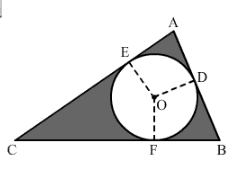Here,

EO = OD (Both the radii)

Because the circle is an incircle, AE and AD are tangents to the circle

$\angle AEO =\angle ADO = 90^{\circ}$

Also,

$\angle A = 90^{\circ}$

Therefore, AEOD is a square.

Thus, we can say that AE = EO = OD = AD = r

CE= CF = 8 – r

BD = DB = 6 – r

CF+ BF = 10

$\Rightarrow$ (8 – r ) + ( 6 – r ) = 10

$\Rightarrow$ 14 – 2r = 10

$\Rightarrow$ r = 2 cm

Area of the shaded portion = Area of the triangle – Area of the circle

$= \left ( \frac{1}{2} \times 6 \times 8 \right ) – \left ( \pi \times 2 \times 2\right )$

= 24 – 12.56 = 11.44 $cm^{2}$

Q.14: In the given figure, $\bigtriangleup ABC$ is right-angled at A. Semicircles are drawn on AB, AC and BC as diameters. It is given that AB = 3 cm and AC = 4 cm. Find the area of the shaded region.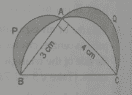Sol:

In triangle ABC, we have

$BC = \sqrt{AB^{2} + AC^{2}}$

$BC = \sqrt{9+ 16}$

$BC = \sqrt{25}$

= 5 cm

Ar( shaded part) = Ar ($\bigtriangleup ABC$ ) + Ar( Semicircle APB) + Ar. (AQC) – Ar. ( Semicircle BAC)

= $\left ( \frac{1}{2} \times 3 \times 4 \right ) + \left ( \frac{1}{2} \pi \times 1.5 \times 1.5 \right ) + \left ( \frac{1}{2} \pi \times 2 \times 2 \right ) – \left ( \frac{1}{2} \pi \times 2.5 \times 2.5 \right )$

$= 6 + \frac{1}{2} \pi \left ( 4 + \frac{9}{4} – \frac{25}{4} \right )$

= 6 + 0 = 6 $cm^{2}$

Q.15: PQRS is a diameter of a circle of radius 6 cm. The lengths PQ, QR and RS are equal. The length PQ, QR and RS are equal. Semicircles are drawn with PQ and QS as diameters, as shown in the given figures. If PS = 12 cm, find the perimeter and area of the shaded region.  [Use $\pi = 1.732$]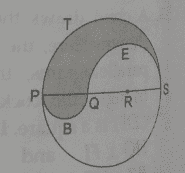Sol:

Perimeter (Circumference of the circle ) = $2 \pi r$

We know:

Perimeter of a semicircle arc = $\pi r$

Now,

For the arc PTS, radius is 6 cm.

Therefore, Circumference of the semicircle PTS = $\pi r = 6 \pi$ cm

For the arc QES, radius is 4 cm.

Therefore, Circumference of the semicircle QES = $\pi r = 4 \pi$ cm

For the arc PBQ, radius is 2 cm.

Therefore, Circumference of the semicircle PBQ = $\pi r = 2 \pi$ cm

Now, perimeter of the shaded region = $6 \pi + 4 \pi + 2 \pi$

= $12 \pi$

$12 \times 3.14$

= 37.68 cm

Area of the semicircle = $= \frac{1}{2} \pi r^{2}$

$= \frac{1}{2} \times 3.14 \times 2 \times 2$

= 6.28 $cm^{2}$

Area of the semicircle PTS = $= \frac{1}{2} \pi r^{2}$

$= \frac{1}{2} \times 3.14 \times 6 \times 6$

= 56.52 $cm^{2}$

Area of the semicircle QES = $= \frac{1}{2} \pi r^{2}$

$= \frac{1}{2} \times 3.14 \times 4 \times 4$

= 25.12 $cm^{2}$

Area of the shaded region = Area of the semicircle PBQ + Area of the semicircle PTS – Area of the semicircle QES

= 6.28 + 56.52 – 25.12 = 37.68 $cm^{2}$

Q.16: The inside perimeter of a running track shown in the figure is 400 m. The length of each of the straight portions is 90 m, and the ends are semicircles. If the track is 14 m wide everywhere, find the area of the track. Also, find the length of the outer boundary of the track.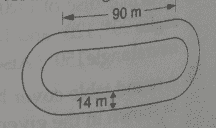Sol:

Length of the inner curved portion = $400 – 2 \times 90 = 220 \; m$

Therefore, Length of each inner curved path = $= \frac{220}{2} = 110 m$

Thus we have:

$\pi r = 110$

$\frac{22}{7} r = 110$

$\Rightarrow r = \frac{110 \times 7}{22} = 35$ m

Outer radius = (35+ 14) = 49 m

Area of track = ( Area of two rectangles [ each $90 \times 14$ m ] + Area of the circular ring with R = 49 m and r = 35 m )

$= \left ( 2 \times 90 \times 14 \right ) + \frac{22}{7} \times \left [ 49^{2} – 35^{2} \right ]$

= 2520 + $\frac{22}{7} \times (2401 – 1225)$

= 2520 + $\frac{22}{7} \times 1176$ = 2520 + 3696 = 6216 $m^{2}$

Length of the outer boundary of the track

= $\left ( 2 \times 90 + 2 \times \frac{22}{7} \times 49 \right )$ = 488 m

Therefore, the length of the outer boundary of the track is 488 m and the area of the track is 6216 sq. m.

Practise This QuestionFind the focal length?